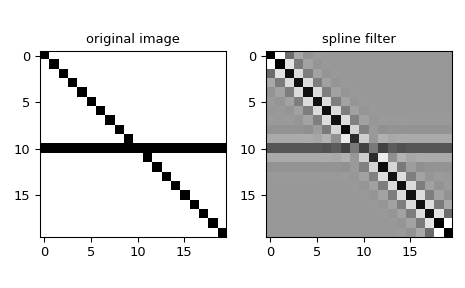# scipy.ndimage.spline_filter¶

scipy.ndimage.spline_filter(input, order=3, output=<class 'numpy.float64'>, mode='mirror')[source]

Multidimensional spline filter.

For more details, see `spline_filter1d`.

`spline_filter1d`

Calculate a 1-D spline filter along the given axis.

Notes

The multidimensional filter is implemented as a sequence of 1-D spline filters. The intermediate arrays are stored in the same data type as the output. Therefore, for output types with a limited precision, the results may be imprecise because intermediate results may be stored with insufficient precision.

For complex-valued input, this function processes the real and imaginary components independently.

New in version 1.6.0: Complex-valued support added.

Examples

We can filter an image using multidimentional splines:

```>>> from scipy.ndimage import spline_filter
>>> import matplotlib.pyplot as plt
>>> orig_img = np.eye(20)  # create an image
>>> orig_img[10, :] = 1.0
>>> sp_filter = spline_filter(orig_img, order=3)
>>> f, ax = plt.subplots(1, 2, sharex=True)
>>> for ind, data in enumerate([[orig_img, "original image"],
...                             [sp_filter, "spline filter"]]):
...     ax[ind].imshow(data, cmap='gray_r')
...     ax[ind].set_title(data)
>>> plt.tight_layout()
>>> plt.show()
```